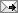## Friday, October 17, 2008

### Wald, LR, and Score Tests

From Cornell, "Econ 620: Three Classical Tests; Wald, LM(Score), and LR tests" is a good description of the Wald, likelihood ratio, and score tests. The Hausman test seems more like an LR test, since it estimates both the restricted and unrestricted equations. I found the statalist post below on the Wald test for exogeneity of regressors:
This test is mentioned along with the theory behind -ivprobit- in Wooldridge's "Econometric Analysis of Cross Section and Panel Data" (2002, pp. 472-477). For the maximum likelihood variant with a single endogenous variable, the test is simply a Wald test that the correlation parameter rho is equal to zero. That is, the test simply asks whether the error terms in the structural equation and the reduced-form equation for the endogenous variable are correlated. If there are multiple endogenous variables, then it is a joint test of the covariances between the k reduced form equations' errors and the structural equation's error. In the two-step estimator, in the second stage we include the residuals from the first-stage OLS regression(s) as regressors. The Wald test is a test of significance on those residuals' coefficients.

Labels:

To view the post on a separate page, click: at (the permalink).